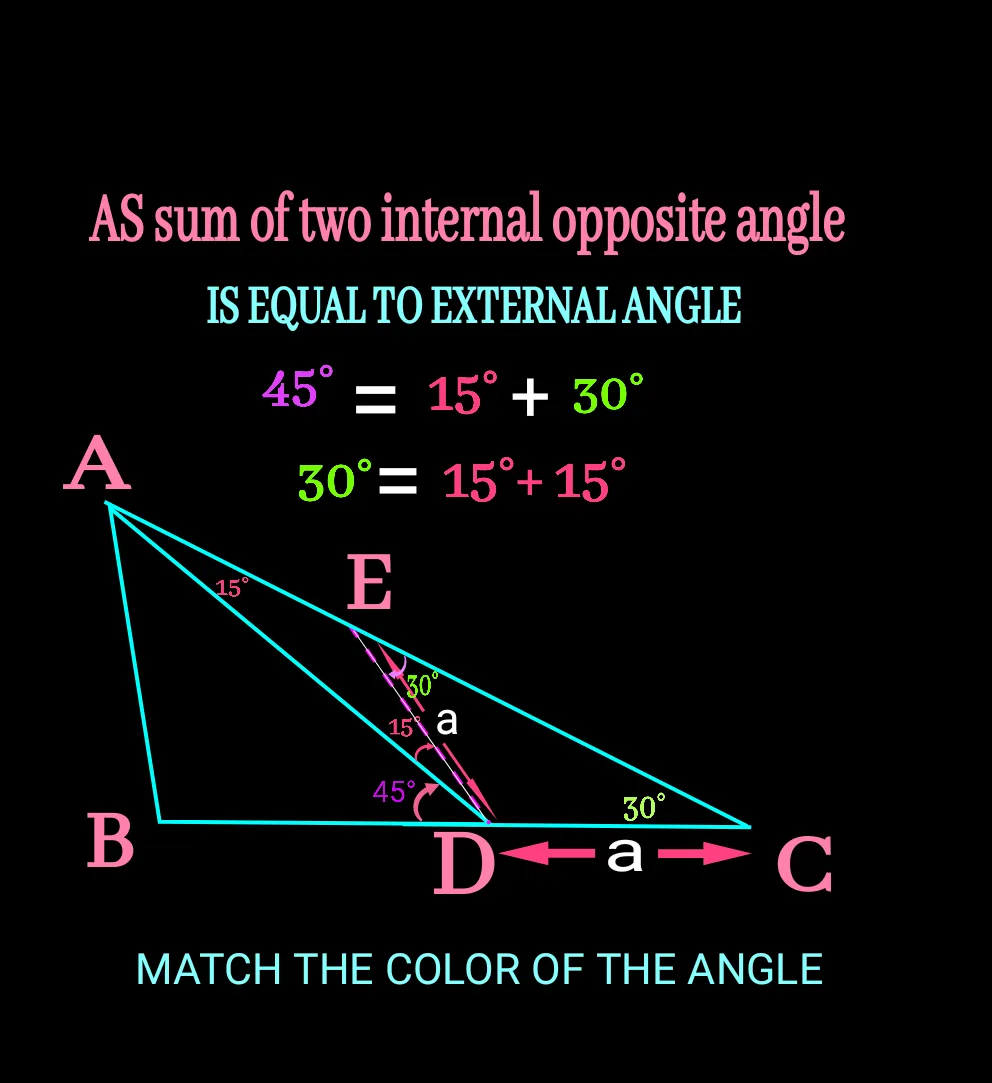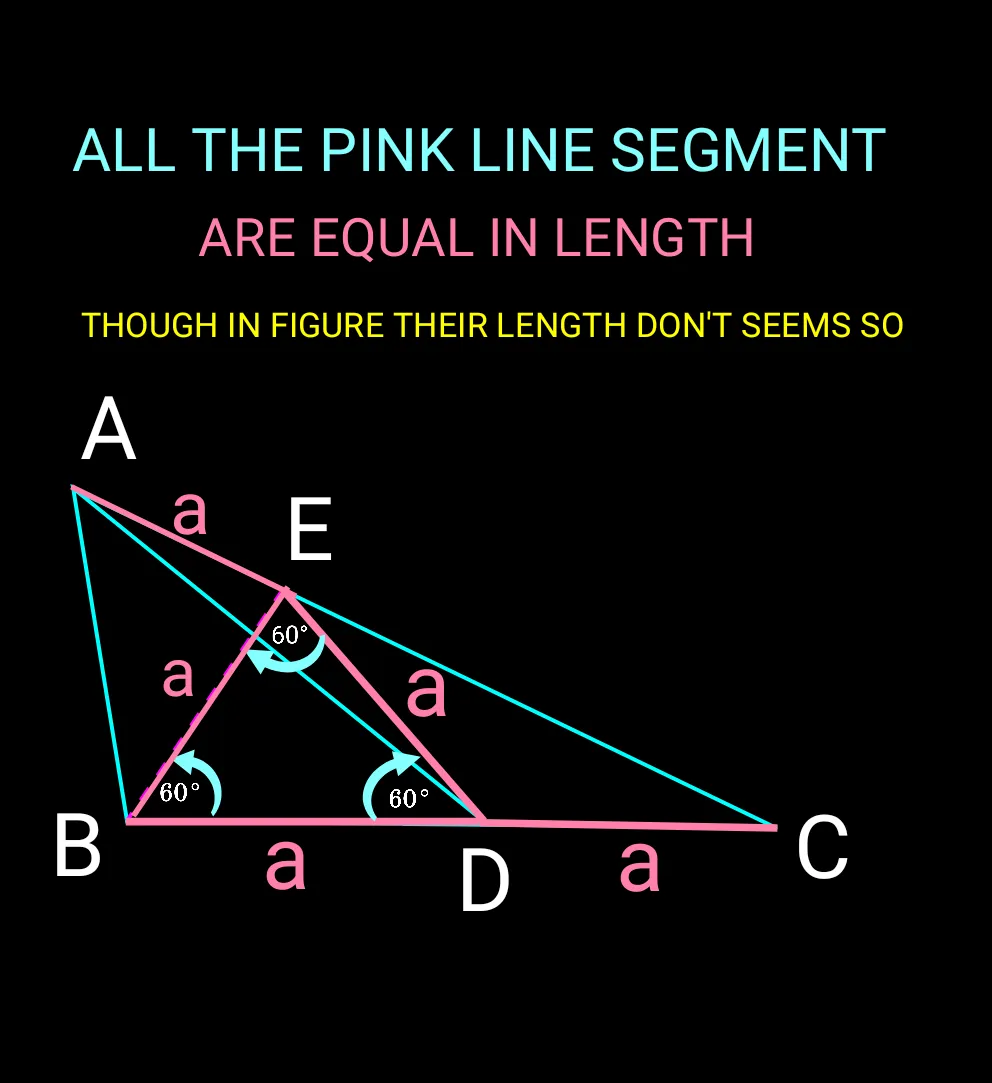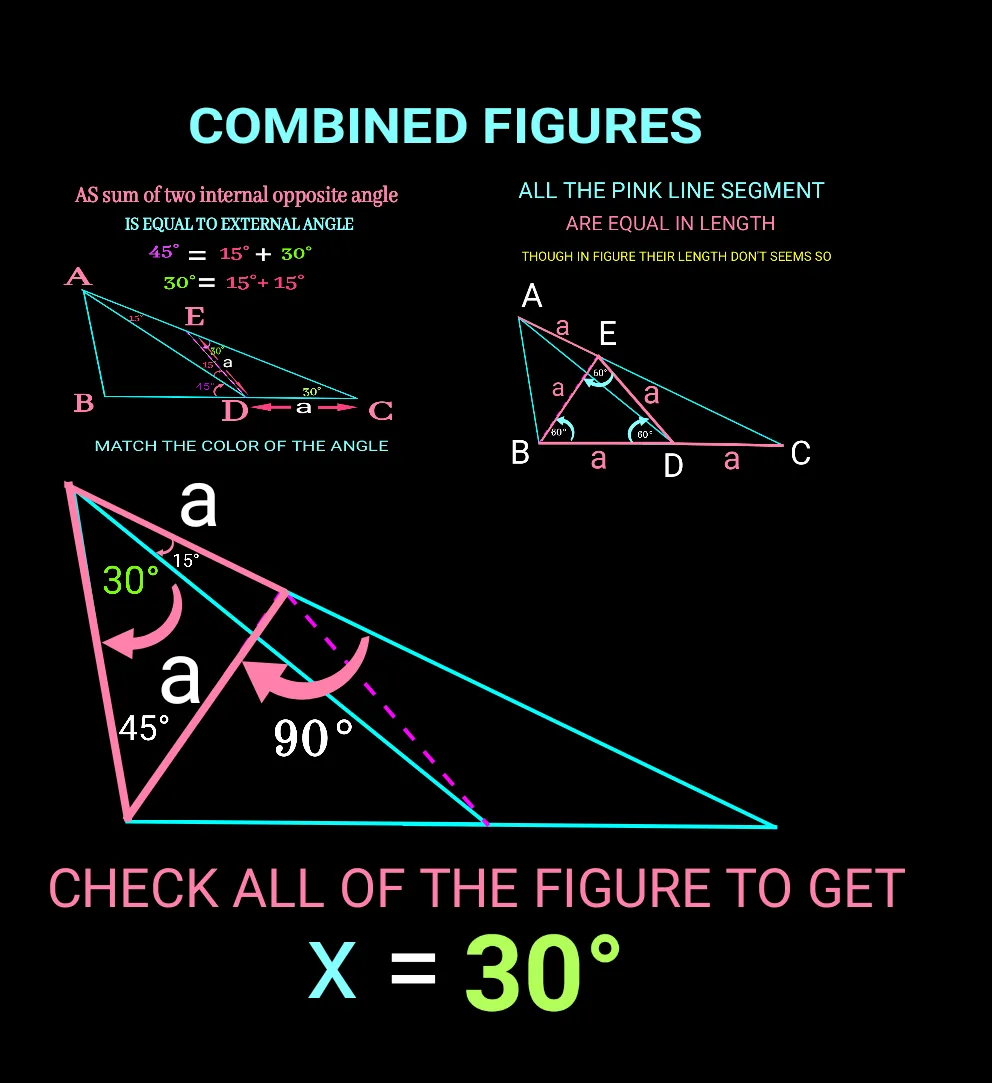# THE EXTERNAL ANGLE OF A TRIANGLE IS SUM OF OPPOSITE INTERNAL ANGLES

Hello maths bugs(🐞) and hivers(🐝)
I hope you are strong and stout and doing good in life.

Once again I have come up with another geometric property.We know that for a triangle any external angle is equal to to sum of opposite internal angles.Today using this problem I wanna solve an interesting problem as you can see in the following figure.To find value of x° our first construction is line segment DE which is equal to DC. So angle DEC and angle ECD are same value of 30° according to construction and given data.Check it in the figure below.To find sum of which two internal angles is equal to which opposite angle use color combination.The only two constructed line segments are shown below:If you use the figure given below , you find ∆BDE an equilateral triangle.Hence all of the angles of it are 60°. To find it we can use angle sum property of a triangle.

Angle BED is equal to 60° as sides of ∆BDE are equal.To reach the final segment of the solution we need to find the value of angle AEB or angle BEC. Both of them are right angle triangle.Angle BEC equal to sum of angle BED and angle DEC. That means 60°+ 30° equal to 90°.Again as angle EAD and angle EDA are equal and the value is 15° and according to the property of the tropic angle ABE equal to angle EAB and both of them is equal to 45°. And the value of x thus 45° minus 15° equals to 30°.. Bravo! Check it below:Here are some of my article which you may have like:

CIR-CUM-CIRCLE

SIMILARITY

IN-CIRCLE

GEO-CENTRE/CENTROID

I hope you enjoyed the solution to find the value of X.

Please stay connected for more article

Thank you for being here

All is well

Regards: @meta007

H2
H3
H4
3 columns
2 columns
1 column